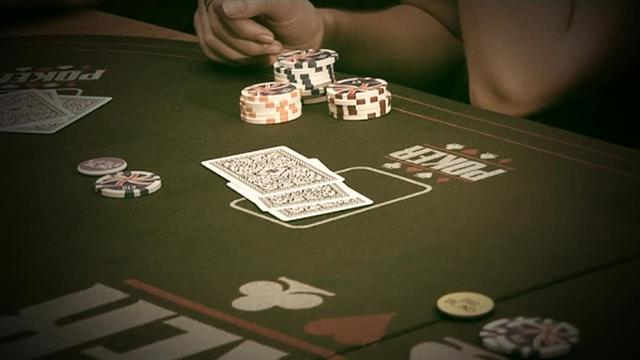Expected Value, Deconstructed

Trying to figure out poker terms? We know just how frustrating that can really be. You think you know a few things and then you wake up to find that you still have quite a bit more of the elephant to eat. The best thing that you can do for yourself is to just keep moving slowly in the direction that you ultimately wish to go. That’s going to be the key to long term success in poker.

We get asked about expected value so much that we might as well talk about it here. In a nutshell, expected value refers to the average outcome of a given scenario over the long term. Note that last phrase: long term. This is not fort short term players. Expected value is all about figuring out the right decision to make over the long run, which is very important when you’re trying to improve your poker game. You have to be able to look at every possible outcome, multiply each by the chance of that outcome taking place, and then adding those numbers together.

You can use a simple six sided dice to look at expected value. If I roll a 1, that has a 1/6 chance of happening. Same with 2 – 6 — all 1/6.

So if I were to multiply each number against the probability, I would have:

1 * 1/6 = 1/6
2 * 1/6 = 2/6
3 * 1/6 = 3/6
4 * 1/6 = 4/6
5 * 1/6 = 5/6
6 * 1/6 = 6/6

You then need to add the probabilities together. If you don’t have a calculator handy, don’t worry — we have you covered there too. The expected value (answer) would be 3.5.But in poker, nothing is that simple. Using the dice metaphor again, what if the die was weighted? This could mean that the number 6 had a 50% chance of coming up more than all of the others. Assuming we hold all of the other numbers static in terms of distribution (an equal chance with all of them except that 6), you would then have:

1 * 1/10 = 1/10
2 * 1/10 = 2/10
3 * 1/10 = 3/10
4 * 1/10 = 4/10
5 * 1/10 = 5/10
6 * 1/2 = 3

That new sum is 4.5

But you might sit and wonder what this has to really do with your poker game. Don’t worry, we’re definitely getting there. Expected value is where the “math” side of poker comes in. If you’re trying to limp with medium pairs, you need to see if it’s going to be worth it. Let’s assume that the pot in question isn’t raised and there are other people that are going to jump in as well. That’s going to be a play that has a good chance of positive expected value.

The poker world can be divided into positive and negative expected value. You want to go with the highest expected value possible for your situation.

If you’re playing Hold’Em, chances are you’ve played these hole cards often: